# Rectangular flowerbed

Around the rectangular flowerbed with dimensions of 5.25 m and 3.50 m, roses should be planted at the same distance from each other so that the roses are located in each corner of the flower bed and are consumed as little as possible. How far do we plant them? How many roses will we need?

d =  175 cm
n =  10

### Step-by-step explanation: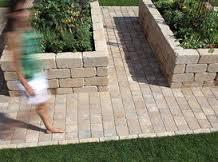Did you find an error or inaccuracy? Feel free to write us. Thank you!Tips to related online calculators
Do you want to calculate greatest common divisor two or more numbers?
Do you want to convert length units?
Do you want to perform natural numbers division - find the quotient and remainder?

#### You need to know the following knowledge to solve this word math problem:

We encourage you to watch this tutorial video on this math problem:

## Related math problems and questions:

• Around the flowerbed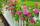Around a rectangular flowerbed with dimensions 5.25 m and 3.5 m are to be planted roses equally spaced so that the roses found in every corner of the flowerbed and consumed them as little as possible. a) At what distance are planted roses? b) How many ros
• Flower bed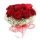The park has a rectangular flower bed with dimensions of 3.2m and 1.5m. How many rose bushes will we plant in the flower bed if 20 dm2 are needed for one bush?
• Florist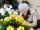Florist has 84 red and 48 white roses. How many same bouquets can he make from them if he must use all roses?
• Gardens colonyGardens colony with dimensions of 180 m and 300 m are to be completely divided into the same large squares of the highest area. Calculate how many such squares can be obtained and determine the length of the square side.
• Gcd and lcm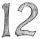Calculate the greatest common divisor and the least common multiple of numbers. a) 16 and 18 b) 24 and 22 c) 45 and 60 d) 36 and 30
• Identical cubesFrom the smallest number of identical cubes whose edge length is expressed by a natural number, can we build a block with dimensions 12dm x 16dm x 20dm?
• Square tilesThe room has dimensions of 12 meters and 5.6 meters. Determine the number of square tiles and their largest dimension to exactly cover the floor.
• Trees in alleyThere are four trees in the alley between which the distances are 35m, 15m and 95m. Trees must be laid in the spaces so that the distance is the same and the maximum. How many trees will they put in and what will be the distance between them?
• Cutting paperDivide a rectangular paper with dimensions 220mm and 308mm into squares of the same size so that they are as large as possible. Specify the length of the side of the square.1st blade 2,5 m, 2nd blade. .1.75 m. How many same long pieces of this two blades can be do the biggest? How long is one piece?
• Glass panelA rectangular glass panel with dimensions of 72 cm and 96 cm will cut the glazier on the largest square possible. What is the length of the side of each square? How many squares does the glazier cut?
• Paving - jointsWe are paving with rectangular pavement 18 cm × 24 cm was placed side by side in height in a row and in the second row in width etc. How many times will the joints meet at a distance 10 m?
• The classroomThe classroom is 9 meters long. The width of the classroom is smaller and can be passed in equally long steps of 55 CM or 70 CM. Determine the width of the classroom.
• Largest squaresHow many of the largest square sheets did the plumber cut the honeycomb from 16 dm and 96 dm?
• Lcm = 22 + gcdThe least common multiple of two numbers is 22 more than their greatest common divisor. Find these numbers.
• TilesHall has dimensions 325 &time; 170 dm. What is the largest size of square tiles that can be entire hall tiled, and how many we need them?
• QuotientFind quotient before the bracket - the largest divisor 51 a + 34 b + 68 121y-99z-33